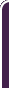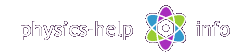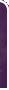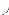homephysics online guidephysics help my info terms of use contactsWhat is Physics? Mechanics Electricity and Magnetism Electric Field Gauss' Law about below subjects Electric Potential Capacity Direct Current Magnetic Field Magnetic Field Laws Magnetic Interactions Electromagnetic Induction Maxwell's Equations Oscillations and Waves Simple Harmonic Motion Damped Harmonic Motion Driven Harmonic Motion Electric Oscillation Alternating Current Wave Motion Elastic Waves Electromagnetic Waves Optics Light Waves Geometrical Optics Interference Polarization Diffraction Fraunhofer Diffraction Dispersion, Absorption, Diffusion Doppler Effect Thermodynamics Ideal Gas Molecular Statistics Transport Phenomena First Law of Thermodynamics Second and Third Laws of Thermodynamics Imperfect Gas Liquids Solids Quantum Physics Thermal Radiation Quantum Properties of Light Wave Properties of Particles Planetary Model of Atom X-Rays Particle in Potential Well Pauli Exclusion Principle Nuclear Physics Solid State Physics Appendices# Translational Dynamics

SI units & Physics constants

Translational dynamics investigates translational motion of objects and deals with effects that forces have on motionHere (all units see here):

m is mass of objectis velocity vectoris linear acceleration vectoris linear momentum vectoris force vector

## General formulas and definition

Definition of mass: mass, m, of a substance or a body is scalar quantity, which defines its inertial and gravitational properties. The unit of mass is kilogram, 1 kg, defined by etalon of mass as specific platinum-iridium cylinder

Linear momentum is vector fefined byForce is vector fefined byResultant force or net force of n forcesapplied to object is defined by vector sumThe polygon rule for net force for case n = 3## Some forces in Mechanics

Gravitational forcewhere g is free-fall acceleration

Frictional forcewhere:is coefficient of friction

N is normal component of total force acting on object from the surfaceSpring forcewhere:

k is spring constant

xo is length of unstretched spring

x lengths of spring stretched by force F

## Newton's laws of motion in inertial reference frames

Inertial reference frame is a frame of reference which is at rest or moving at a constant velocity, which obeys the Newton's First Law

Newton's First Law

Ifthen, that is the object is in uniform motion, or at rest

Newton's Second Law:

- for general case- for constant massNewton's Third Lawwhere:is force on mass m1 applied by mass m2, andis force on mass m2 applied by mass m1, shown in the below diagram## Law of conservation of linear momentum

Total linear momentum of system of n particles is defined by vector sumwhereis linear momentum of i-th particle with massand velocityThe law of conservation of linear momentum

Ifthenwhereis resultant force of all external forces applied to the system of particles

Totally inelastic collision is the collision after which the objects are moving together as one bodyThe velocity after inelastic collisionTotally elastic collision is the collision when total machanical energy conserves in the collision

Collision for unidirectional motion:The velocities of objects after collision:whereis coefficient of restitution

For totally elastic collisionFor totally inelastic collision## Impulse-Momentum Theorem

Impulse of forcewhereis average force acting on object during time intervalgiven by integralImpulse-Momentum theoremwhereandare initial and final linear momentums of the object respectively

Motion of center of mass of system of particles

The position vector of center of mass of n particles:where:andare mass and radius vector of position of i-th particleis total mass of the system of particles

The velocity vector of center of massThe Newton's Second Law for motion of center of masswhereis resultant force of all external forces applied to the system of particles

## Propulsion motion of object with variable massWhere:

m is current mass of objectis current velocity of objectis trust (or reactive force)is velocity of ejected mass with respect to the object

The thrust forcewhereis rate of change of mass m

The equation for motion of object with variable massCurrent speed of object with mass m starting from rest with original masshome  |  physics online guide  |  physics help  |  my info  |  terms of use  |  contacts Dr. Nikitin © 2010